• 在用生成对抗网络生成二维数据点的时候遇到代码里的一个问题，就是numpy中的一维线性插值函数interp到底是怎么用的，在这个上面费了点功夫，因此现将其用法给出。 在生成对抗网络的二维样本生成的例子中，涉及了...

前言：
在用生成对抗网络生成二维数据点的时候遇到代码里的一个问题，就是numpy中的一维线性插值函数interp到底是怎么用的，在这个上面费了点功夫，因此现将其用法给出。
在生成对抗网络的二维样本生成的例子中，涉及了一维线性插值，代码里使用的是：

numpy.interp(x, xp, fp, left=None, right=None, period=None)
上网查了百度和谷歌发现都没有具体的中文的解释，只有官方的英文解释：

$$One-dimensional$$ $$linear$$ $$interpolation.$$ $$Returns$$ $$the$$ $$one-dimensional$$ $$piecewise$$ $$linear$$ $$interpolant$$ $$to$$ $$a$$ $$function$$ $$with$$ $$given$$ $$values$$ $$at$$ $$discrete$$ $$data-points.$$
官方给出的例子如下：

 >>> xp = [1, 2, 3]
>>> fp = [3, 2, 0]
>>> np.interp(2.5, xp, fp)
1.0
>>> np.interp([0, 1, 1.5, 2.72, 3.14], xp, fp)
array([ 3. ,  3. ,  2.5 ,  0.56,  0. ])
>>> UNDEF = -99.0
>>> np.interp(3.14, xp, fp, right=UNDEF)
-99.0

Plot an interpolant to the sine function:

>>> x = np.linspace(0, 2*np.pi, 10)
>>> y = np.sin(x)
>>> xvals = np.linspace(0, 2*np.pi, 50)
>>> yinterp = np.interp(xvals, x, y)
>>> import matplotlib.pyplot as plt
>>> plt.plot(x, y, 'o')
[<matplotlib.lines.Line2D object at 0x...>]
>>> plt.plot(xvals, yinterp, '-x')
[<matplotlib.lines.Line2D object at 0x...>]
>>> plt.show()      
其中对于第一例子，只要画出图像就很好理解了：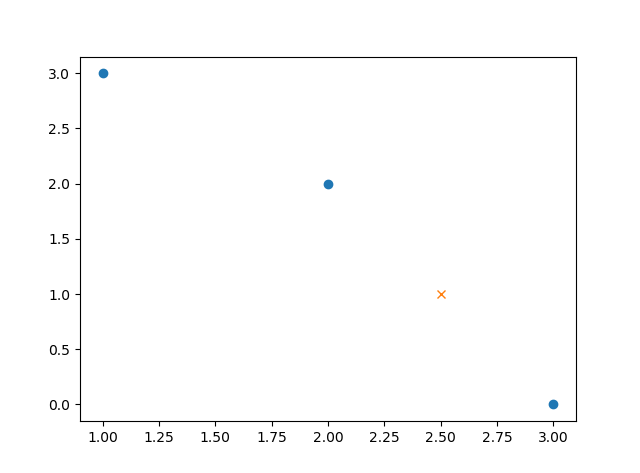也就是说只要参数中的$$2.5$$是我们要插入的值，我们要做的是连接$$(2,2)$$和$$(3,0)$$这两个点，然后在$$x=2.5$$这里做垂线，那么相交的那个点(也就是$$(2.5,1.0)$$这个点)就是我们要插入的点了。

转载于:https://www.cnblogs.com/Stoner/p/9141464.html
展开全文• 更多编程教程请到：菜鸟...友情链接： ... 直接列出函数： numpy.interp(x, xp, fp, left=None, right=None, period=None) x - 表示将要计算的插值点x坐标 xp - 表示已有的xp数组 fp - 表示对应于已有的xp数组的值 lef
更多编程教程请到：菜鸟教程 https://www.piaodoo.com/
友情链接：
高州阳光论坛https://www.hnthzk.com/
人人影视http://www.sfkyty.com/
直接列出函数：

numpy.interp(x, xp, fp, left=None, right=None, period=None)

x - 表示将要计算的插值点x坐标
xp - 表示已有的xp数组
fp - 表示对应于已有的xp数组的值
left - 表示当x值在xp中最小值左边时，x对应y的值为left
right - 表示当x值在xp中最大值右边时，x对应y的值为right
（left和right表示x在xp的域外时，y的取值）
example：
1.

import numpy as np
xp = [1, 2, 3]
fp = [3, 2, 0]
print(np.interp(2.5, xp, fp))

output：1.0
2.

import numpy as np
xp = [1, 2, 3]
fp = [3, 2, 0]
x = [0, 1, 1.5, 2.72, 3.14]
print(np.interp(x, xp, fp))

output：[3. 3. 2.5 0.56 0. ]
3.

import numpy as np
xp = [1, 2, 3]
fp = [3, 2, 0]
x = [0, 1, 1.5, 2.72, 3.14]
print(np.interp(x, xp, fp, -99, 99))

output：[-99. 3. 2.5 0.56 99. ]
补充知识：numpy 的一维插值函数interp

numpy.interp(x, xp, fp, left=None, right=None, period=None)

返回离散数据的一维分段线性插值结果，浮点数或复数（对应于fp值）或ndarray. 插入数据的纵坐标，和x形状相同。
x: 数组，待插入数据的横坐标.
xp: 一维浮点数序列，原始数据点的横坐标，如果period参数没有指定那么就必须是递增的。否则，在使用xp = xp % period正则化之后，xp在内部进行排序.
fp: 一维浮点数或复数序列原始数据点的纵坐标，和xp序列等长.
left: 可选参数，类型为浮点数或复数（对应于fp值），当x < xp时的插值返回值，默认为fp.
right: 可选参数，类型为浮点数或复数（对应于fp值），当x > xp[-1]时的插值返回值，默认为fp[-1].
period: None或者浮点数，可选参数. 横坐标的周期. 此参数使得可以正确插入angular x-coordinates. 如果该参数被设定，那么忽略left参数和right参数。
插入横坐标在原函数横坐标范围内

x = 2.5     # 要插入值的横坐标
xp = [1, 2, 3]   # 要插入序列的横坐标
fp = [3, 2, 0]   # 要插入序列的纵坐标
y = np.interp(x, xp, fp) # 返回插入值的纵坐标 1.0
plt.plot(xp, fp, '-o')
plt.plot(x, y, 'x')
plt.show()

插入横坐标在原函数横坐标外（默认）

x = [0, 1, 1.5, 2.72, 3.14] # 左侧外部默认为原函数最左侧函数值，右侧默认为右侧
xp = [1, 2, 3]
fp = [3, 2, 0]
y = np.interp(x, xp, fp) # array([ 3. ,3. ,2.5 ,0.56, 0. ])
plt.plot(xp, fp, '-o')
plt.plot(x, y, 'x')
plt.show()

插入横坐标在原函数横坐标外（指定）

x = 3.14
xp = [1, 2, 3]
fp = [3, 2, 0]
UNDEF = -99.0
y = np.interp(x, xp, fp, right=UNDEF) # -99.0
plt.plot(xp, fp, '-o')
plt.plot(x, y, 'x')
plt.show()

正弦插值

x = np.linspace(0, 2 * np.pi, 10) # 在0到2pi的范围内均匀取10个点
y = np.sin(x)      # sin函数x横坐标对应的y值
xvals = np.linspace(0, 2 * np.pi, 50) # 均匀取50个
yinterp = np.interp(xvals, x, y) # 在映射关系为y的x中插入xvals
plt.plot(x, y, 'o')
plt.plot(xvals, yinterp, '-x')
plt.show()

以上这篇Numpy一维线性插值函数的用法就是小编分享给大家的全部内容了，希望能给大家一个参考，也希望大家多多支持菜鸟教程www.piaodoo.com。


展开全文• 三线性插值（trilinear interpolation）主要是用于在个3D的立方体中，通过给定顶点的数值然后计算立方体中其他点的数值的线性插值方法。 具体推导过程见参考资料1，这里直接给出最终公式： 其中，坐标(x,y,z...
三线性插值（trilinear interpolation）主要是用于在一个3D的立方体中，通过给定顶点的数值然后计算立方体中其他点的数值的线性插值方法。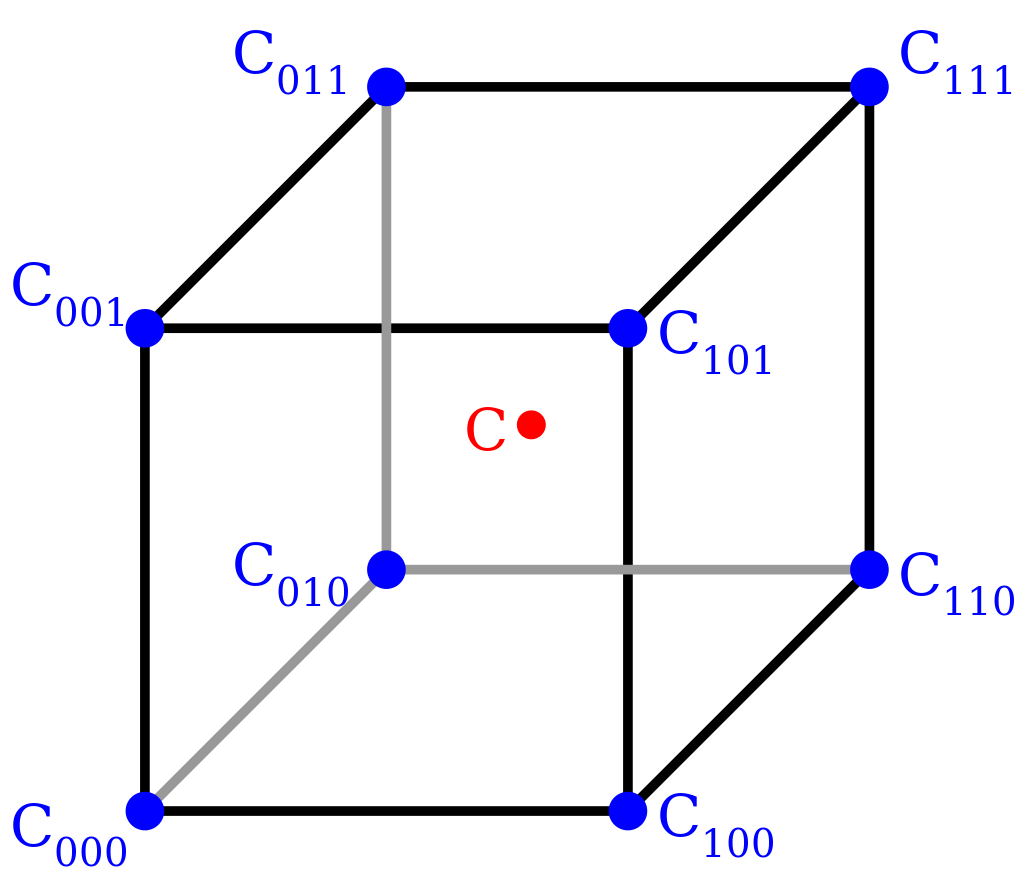具体推导过程见参考资料1，这里直接给出最终公式：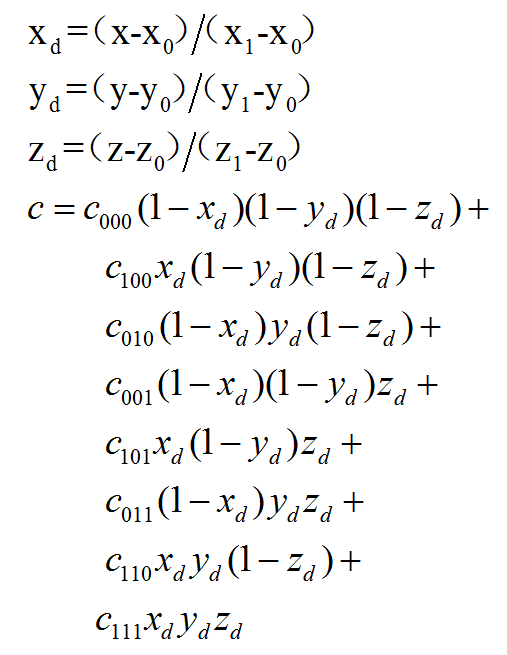其中，坐标(x,y,z)为c，(x0,y0,z0)为坐标相对最小的点。
参考资料

Trilinear interpolation


展开全文线性插值 三线性插值
• 按照公式yi = y1 +（y2-y1）/（x2-x1）*（xi-x1），使用'x'和'y'对'xi'点进行一维线性插值，得到'yi'。 变量： - 'x' 是列向量 [mx 1]，单调递增。 -“ y”是矩阵[mxn]，对应于“ x”。 - 'xi' 是列向量 [px 1]，...matlab
• interpl( )一维插值函数 格式： yi＝interpl (x, y, xi, 'method') 功能：为给定的数据对(x,y)以及x坐标上的插值范围向量xi，用指定所使用的插值方法method实现插值。yi是插值后的对应数据点集的y坐标 简单来...
什么是插值？

正常我们进行数据处理时，是希望得到一个线性的数据，但是在数据极少的情况下，数据不足以支撑分析的进行，对已知的函数点进行数据、模型的处理和分析，这时就需要使用一些数学的方法，产生一些新的但又比较靠谱的值来满足分析需求。我们去调用MATLAB的一些库函数来实现，这个功能就是“插值”

插值法：
插值法又称“内插法”，是利用函数f (x)在某区间中已知的若干点的函数值，作出适当的特定函数，在区间的其他点上用这特定函数的值作为函数f (x)的近似值，这种方法称为插值法。如果这特定函数是多项式，就称它为插值多项式。
简单说：就是根据已知数据点（条件），来预测未知数据点值得方法
比方说你知道了前五年的人口数量和增长率，要求预测未来五年的人口数量，这时便需要一个
interpl( )一维插值函数
格式：
yi＝interpl (x, y, xi, 'method')

功能：为给定的数据对(x,y)以及x坐标上的插值范围向量xi，用指定所使用的插值方法method实现插值。yi是插值后的对应数据点集的y坐标
简单来说：已知样本点坐标x,y，求xi处的函数值yi，插值方法是method。
也就是我们所说的函数方程的求解
插值的方法method有以下5种可供选择：
nearest(最邻近插值法)
linear(线性插值)
spline(三次样条插值)
cubic(三次立方插值)
pchip(三次Hermite插值）
默认为 linear(线性插值)
常用的为’spline’和’cubic’
举例：
x=0:20;
y=x.*sin(x);
x1=0:0.25:10;
y1=interp1(x,y,x1);
plot(x,y,'kd',x1,y1)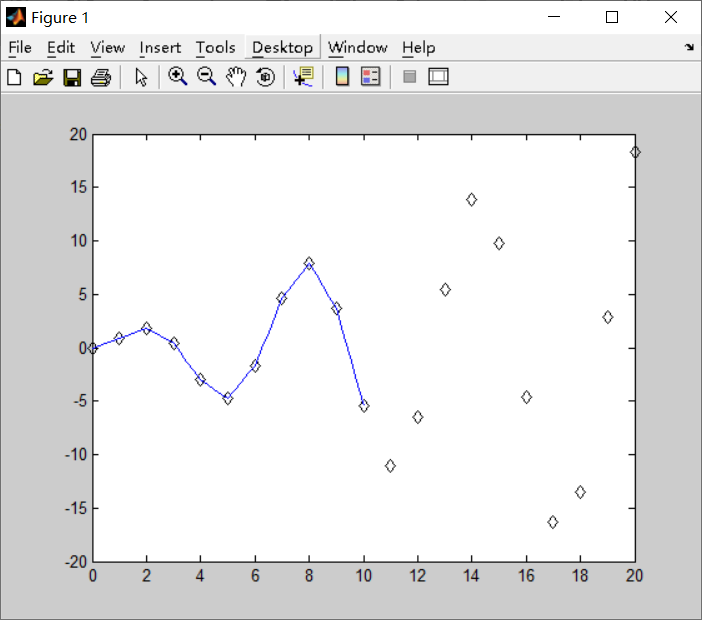四种插值方法比较：
x=0:20;
y=x.*sin(x);
x1=0:0.25:20;

%分段线性插值
y1=interp1(x,y,x1);
figure
subplot(2,2,1)
plot(x,y,'kd',x1,y1,'r')
title('分段线性插值')

%最邻近插值法nearest
y2=interp1(x,y,x1,'nearest');
subplot(2,2,2)
plot(x,y,'kd',x1,y2,'r');
title('最邻近插值法nearest')

%三次样条插值spline
y3=interp1(x,y,x1,'spline');
subplot(2,2,3)
plot(x,y,'o',x1,y3,'r')
title('三次样条插值spline')

%立方插值法
y4=interp1(x,y,x1,'cubic');
subplot(2,2,4)
plot(x,y,'o',x1,y4,'r');
title('立方插值cubic')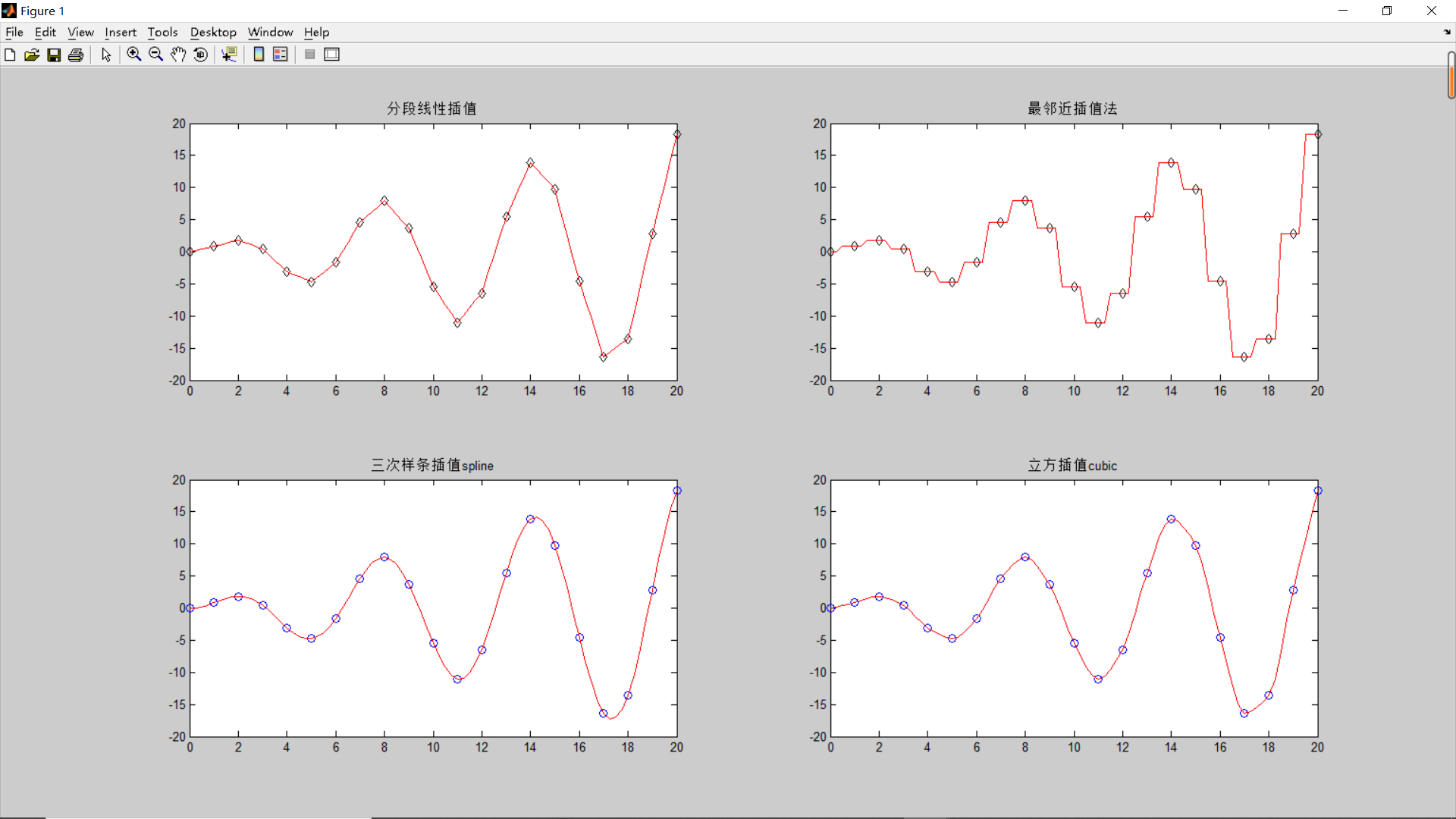由上图可以看出：
这几种方法在速度、平滑性、内存使用方面有所区别，在使用时可以根据实际需要进行选择。包括：
1最临近插值是最快的方法，但是使用它得到的结果平滑性最差。
2线性插值要比最邻近插值占用更多的内存，运行时间略长。与最近邻插值不同，它生成的结果是连续的，但在顶点处会有坡度变化。
3三次多项式插值需要更多的内存，而且运行时间比最邻近法和线性插值要长。但是，在使用此法时，插值数据及其导数都是连续的。
4立方插值的运行时间相对来说最长，内存消耗比三次插值略少。它生成的结果平滑性最好。
1 linear(线性插值)
线性插值
线性插值是利用斜率曲线对一个或多个自变量和因变量之间关系进行建模的一种回归分析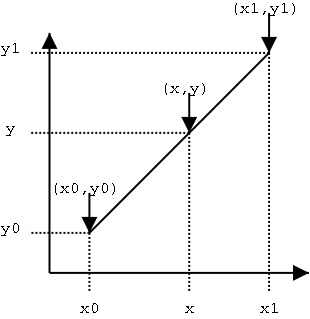线性插值是用一系列首尾相连的线段依次连接相邻各点，每条线段内的点的高度作为插值获得的高度值。
我们知道其斜率是相等的
也就是：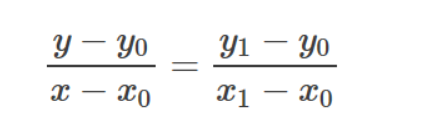以(x0,y0)表示某条线段的前一个端点，(x1,y1)表示该线段的后一个端点，则对于在[x0,x1]范围内的横坐标为x的点，其高度y为：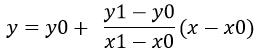化简后最后公式的表示：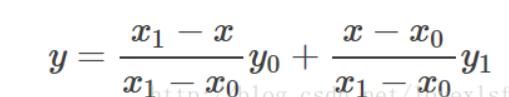2 最邻近插值法
就是根据邻近的两个数值，计算其平均值，然后根据数值的缩放倍数，
四舍五入选取最接近的整数
这样的做法就会导致数据的变化不连续，
Cubic立方插值
如果想要保证各段曲线连接处光滑（一阶导数相同），并且不想使用除法运算，可以考虑Cubic插值函数：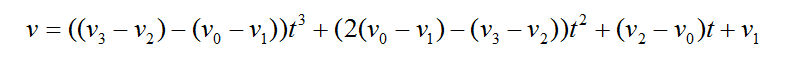其中，v代表插值点，v0、v1、v2、v3代表4个连续的节点。t取值为[0,1]，将会产生一段连接v1和v2的曲线。也就是说，如果有n个节点，Cubic插值函数将会产生(n-2)段曲线，位于首尾两端的节点不会纳入曲线。
4三次样条插值spline
用分段三次多项式曲线光滑地连接相邻样本点，整体上具有函数、一阶和二阶导数连续性
具体请参看
三次样条插值 cubic spline interpolation
interp2
功能 二维数据内插值（表格查找）
格式
ZI = interp2(X,Y,Z,XI,YI)

这里X,Y,是已有X，和Y的坐标，Z是表示Z=(X,Y)以为x行y为列的矩阵     X,Y,Z是同维数矩阵表示网格数据
XI 和 YI 是查询点的坐标,ZI是同维数矩阵表示插值点.
简单说：
ZI=interp2(x,y,z,xi,yi)其中,x,xi为行向量,y,yi为列向量.  Z为x行y列的矩阵
本质也就是在 X等于某个值 Y等于某个值的情况下 求该点的Z值
我们举个例子：
行3-D绘图操作时，涉及到x、y、z三组数据，而x、y这两组数据可以看做是在Oxy平面内对坐标进行采样得到的坐标对（x，y）
那么我们现在采集9个点，(x,y)  就可以知道对应点的Z 的值
  (0,0),(1,9),(2,0);

(0,1),(1,1),(2,1);

(0,2),(1,2),(2,2);

把个点的x坐标单独列出来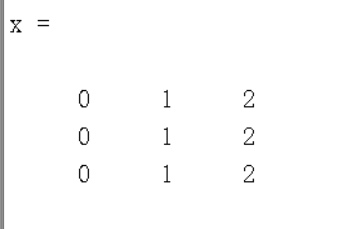再把个点的Y坐标单独列出来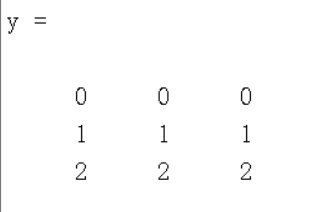那么九个坐标点对应的Z值为：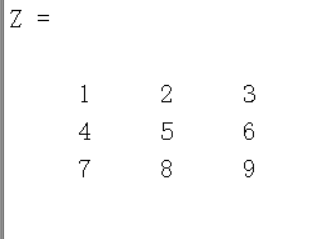也就是本质就是  X存储行  Y存储列  而Z为对应位置的值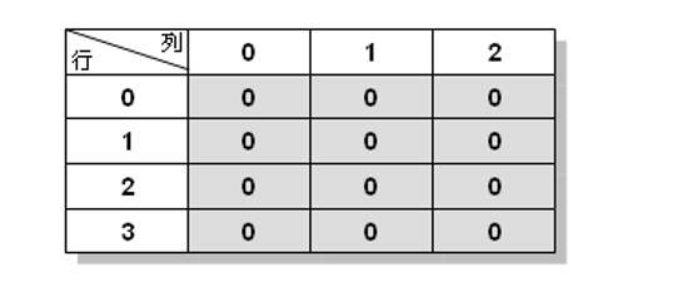返回矩阵ZI：
其元素包含对应于参量XI 与YI（可以是向量、或同型矩阵） 的元素，
即Zi(i,j) ==[Xi(i,j),yi(i,j)]。
若Xi与Yi 中有在X 与Y范围之外的点，则相应地返回nan（Not a Number）
二维线性插值与一维线性插值插值法相同,同为以下几种
ZI = interp2(X,Y,Z,XI,YI,method)
用指定的算法method 计算二维插值：
’linear’：双线性插值算法（缺省算法）；
’nearest’：最临近插值；
’spline’：三次样条插值；
’cubic’：双三次插值。
我们还是来举一个例子
meshgrid函数：
用法：  [X,Y]=meshgrid(x,y)
简单地说，就是产生Oxy平面的网格坐标。
[X,Y]=meshgrid(0:2,0:2)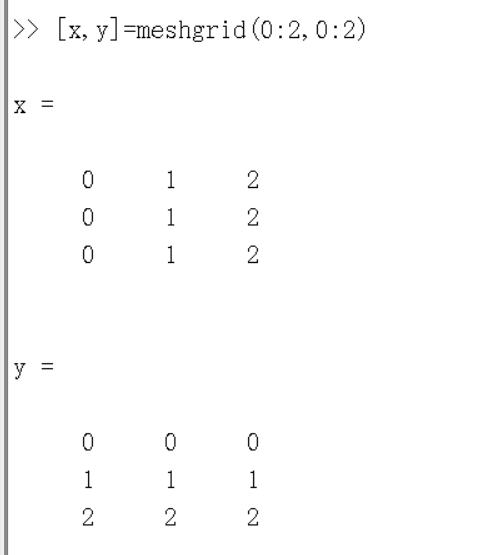将行坐标和列坐标分开存储再两个矩阵当中，
x、y结合，便表示了一个坐标矩阵。meshgrid就是产生这样两个矩阵
那么我们来看下面的代码：
[x,y]=meshgrid(0:2,0:2)
z = [1:3;4:6;7:9]
surf(x,y,z)
[x2,y2]=meshgrid(1:0.1:2,0:0.1:2)
z1 = interp2(x,y,z,x2,y2,'cubic');
surf(x2,y2,z1)

其中x 和 y 的范围为 0：2   间隔为1       已知9个z值数据
那么 x2 和 y2  的取值 就要在0 和2之间  否则就会返回nan（Not a Number）
所以定义x2和y2 的范围为 0：2   间隔为0.1   求在该范围内对应z1的值
插值前的图像坐标及对应值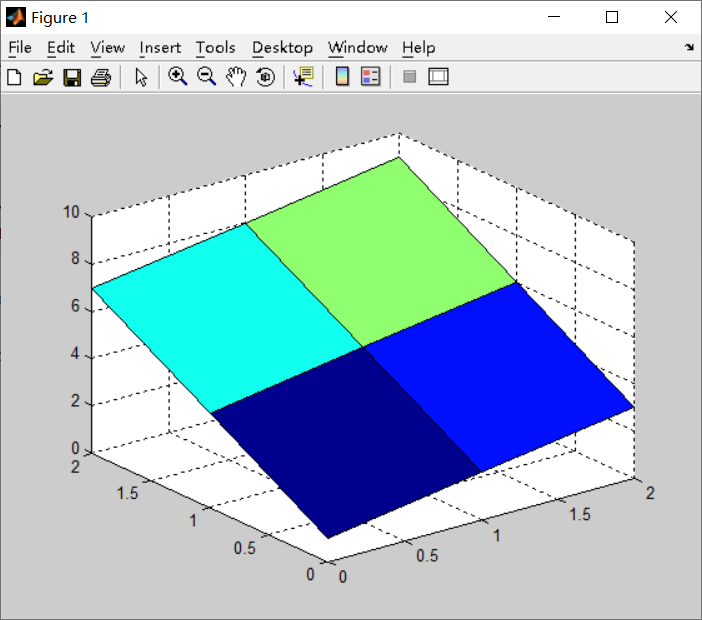只有 0，1，2 处有对应数值
插值后的图像坐标及对应值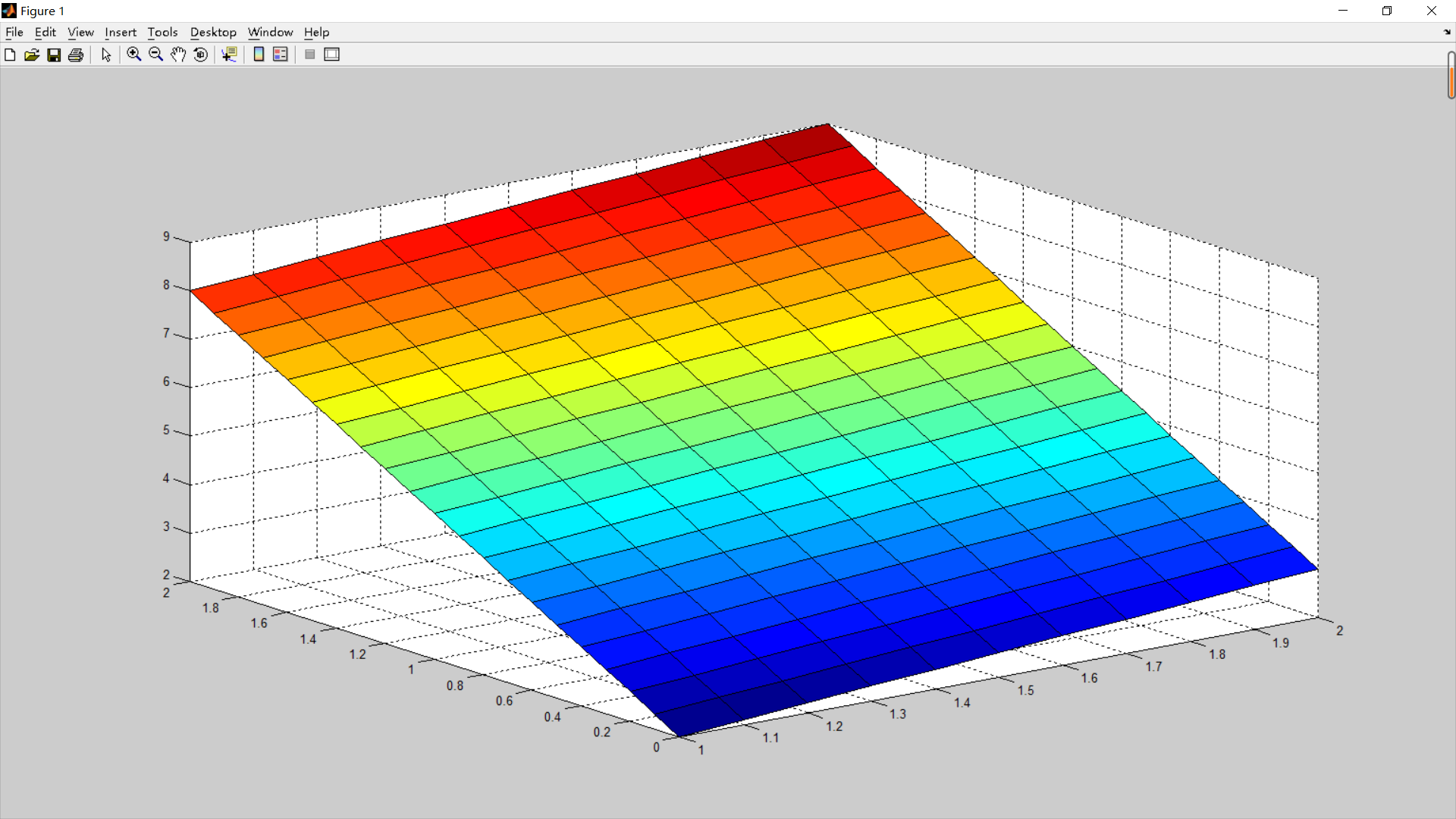展开全文interp1 interp2 matlab 插值函数
• 在理解一维线性插值的基础上，我们可以去构建更高维度的插值算法。原理假设我们已知坐标(x0,y0)(x_0,y_0)与(x1,y1)(x_1,y_1)，要得到[x0,x1][x_0,x_1]区间内某一位置 xx 在直线上的值。根据图中所示，我们得到 y...c语言
• 今天在阅读大牛代码的时候，发现了Linear Interpolation一次，百度之，学习之，记录于此。1.关于插值：插值，是根据已知的数据序列(可以理解为你坐标中一系列离散的点)...线性插值线性插值是针对一维数据的插值方...
• 数学上定义:线性插值是指插值函数为次多项式的插值方式,其在插值节点上的插值误差为0; 在图片上，我们利用线性插值的算法，可以减少图片的锯齿,模糊图片; 线性插值的计算规则 假设我们已知坐标 (x0, y0) 与 ...
• 插值：求过已知有限个数据点的近似函数。 拟合：已知有限个数据点，求近似函数，不要求过已知数据点，只要求在某种意义下它在这些点...下面介绍几种基本的、常用的插值：拉格朗日多项式插值、牛顿插值、分段线性插...
• 表格线性插值 ** ** 博客背景简述 ** 在做项目时需要导入如上图CSV表格，利用线性插值进行查表，欲求出中间方框标志的那个数 代码部分 #导入所需的库 import numpy as np import pandas as pd def Look_Up(x_...python csv
• ## 图像处理之双线性插值法

万次阅读 多人点赞 2018-04-22 19:02:31
1、线性插值的解释 单线性插值法 双线性插值法 2、另位牛人讲的比较易懂 1.双线性插值 2.存在的问题 3、又是另位讲的通俗易懂 1，原理 2，计算方法 3，加速以及优化策略 3.1 源图像和目标图像几何中心的对齐...
• 线性插值 先介绍线性插值的概念。 已知两个点(x1, y1)、(x2, y2)，求它们中间横坐标为x的点的y值。 则可以利用如下公式进行插值计算。其中a和(1-a)为x距离x1和x2的距离占(x2-x1)的比例。 y = a*y1 + (1-a)*y2...数字图像处理
• 本文简述了种三角形线性插值的方法(本文仅讨论二情况) 相关问题 给定个三角形的顶点坐标(P0, P1 和 P2)以及对应的数值(V0, V1 和 V2),插值求解三角形内一点(坐标给定为P)的数值(V). 问题说的有些抽象,给...数学 三角形 重心坐标
• 关于线性线性插值函数 自己下来看看吧。。。还是很不错的 是个作业
• 3.1 一维插值方法 1、数据处理的应用背景 2、插值的基本原理 3、引例 3.1、引例演示 4、一维插值定义 5、一维插值原理 6、一维插值方法 6.1、拉格朗日插值 6.2、分段线性插值 6.3、三次样条插值 3.2 二维插值方法 1...数学建模 matlab 数据拟合
• MATLAB 提供了 interp1(x,y,xq,'Method')函数命令可以进行一维插值，其中一维插值有四种常用的方法，也就是 ‘Method’ 可以选择邻近点插值Nearest，线性插值Linear，三次样条插值Spline和立方插值Pchip。...matlab 数学建模
• 3D图像线性插值算法实现 三线性插值原理 三线性插值原理与双线性插值原理类似，双线性插值是根据周围四个点计算得到新的插值点，三线性插值是对个立方体中周围8个顶点进行计算。 1）首先在x轴上进行插值，根据...python
• 作为图像放缩 (Scale) 的手段，常见的传统插值方法有：最近邻插值 (Nearest Neighbour Interpolation)、线性插值(Linear Interpolation)、双线性插值 (Bilinear Interpolation)、双三次插值 (Bicubic interpolation)...
• 线性插值一次为：0，5，10，15，20，25，30，35，40 即认为其变化（增减）是线形的，可以在坐标图上画出条直线 在数码相机技术中，这些数值可以代表组成一张照片的不同像素点的色彩、色度等指标。 为了方便理解...数学 图像处理
• 先讲一下线性插值：已知数据 (x0, y0) 与 (x1, y1)，要计算 [x0, x1] 区间内某位置 x 在直线上的y值（反过来也是一样，略）：   y−y/0x−x0=y1−y0/x1−x0 y−y0/x−x0=y1−y0/x1−x0     y...
• 线性插值作为种滤波器，广泛应用在信号处理，数字图像和视频处理等应用中。 中文名 双线性插值 外文名 Bilinear Interpolation 介绍 双图像缩放
• 文章目录、基础概念插值是什么拟合是什么插值和拟合的相同点插值和拟合的不同点二、常用的基本插值方法高次多项式插值拉格朗日多项式插值牛顿插值差商矩阵低次多项式插值（不易震荡）分段线性插值Hermite插值三次...matlab
• 线性插值 如果你只处理分离的数据、想知道分离点之间的某些值，需要用到某种类型的插值。这种情况如图5-17坐标所示。对某些分离的(整数) X值，你知道Y值。当X=2，你知道Y=10，X=3时Y=30。但你不知道X=2.7时的Y值。
• ## 线性插值算法

千次阅读 2017-10-27 17:03:47
里面用到了图像的单应性矩阵变换，在最后的图像重映射，由于目标图像的坐标是非整数的，所以需要用到插值的方法，用的就是双线性插值，下面的博文主要是查看了前辈的博客对双线性插值算法原理进行了个总结，在这里...
• 通常，数据是在常规网格上指定的（值写在2D或3D网格的顶点位置）或在线上（在一维的情况下），但是程序需要在该网格上的随机位置求值。如果样本位于网格顶点上，那么我们可以简单地使用存储在网格顶点上的值。但是，...可视化
• 1.为什么要用图像的插值？ 在图像的放大和缩小的过程中，需要计算新图像像素点对应原图的位置，如果计算的位置不是整数，就需要用到图像的内插，我们需要寻找在原图中最近的像素点赋值给新...2.一维线性插值 ......